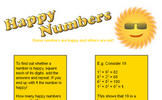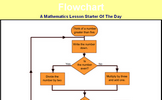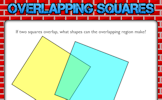# Show that,,,

### Term 2 starting in week 11 :: Estimated time: 2 weeks

• "Show that" with number
• "Show that" with algebra
• "Show that" with shape
• "Show that" with angles
• "Show that" with data
• "Show that" with congruent triangles

For higher-attaining pupils:

• "Show that" with vectors
• Formal proof with congruent triangles

This page should remember your ticks from one visit to the next for a period of time. It does this by using Local Storage so the information is saved only on the computer you are working on right now.

## Lesson Starters

Here are some suggestions for whole-class, projectable resources which can be used at the beginnings of each lesson in this block.

### 1st Lesson#### Cracked Clock

How did the clock break if the numbers on each of the pieces added up to the same total?

### 2nd Lesson#### Don't Touch!

A number placing puzzle which, when solved, raises the notion of proof.

### 3rd Lesson#### Happy Numbers

To find out whether a number is happy or not, square each of its digits, add the answers and repeat. If you end up with 1 the number is happy! How many other happy numbers can you find?

### 4th Lesson#### Flowchart

Use the flowchart to generate a sequence of numbers. Which number will reach 1 the fastest?

### 5th Lesson#### Tindice

How can you put the dice into the tins so that there is an odd number of dice in each tin?

### 6th Lesson#### Overlapping Squares

If two squares overlap, what shapes can the overlapping region make?

Some of the Starters above are to reinforce concepts learnt, others are to introduce new ideas while others are on unrelated topics designed for retrieval practice or and opportunity to develop problem-solving skills.

White Rose ResourcesEnd of block assessments provide a quick progress check at the end of each block of learning to make sure students have understood the content covered. This Scheme of Learning was produced by White Rose Maths and is used here with permission granted on 30th June 2021.For All: